# Mathematics - Signals And Systems - Sinusoidal and Complex Exponential Signals.[Image1]

## Introduction

Hey it's a me again @drifter1!

Today we continue with my mathematics series about Signals and Systems in order to cover Sinusoidal and Complex Exponential Signals.

So, without further ado, let's get straight into it!

## Sinusoidal Signals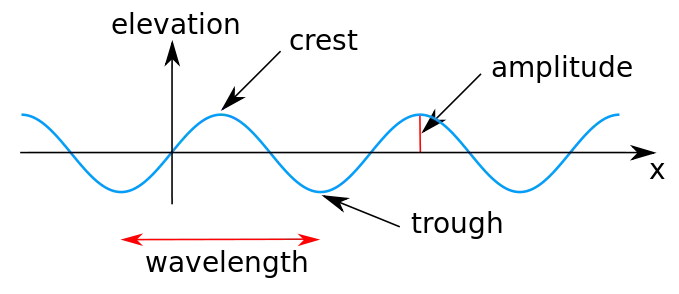[Image 2]

Sinusoidal signals are periodic signals, which are based on the trigonometric functions sine (sin) and cosine (cos).

### Continuous-Time Sinusoidals

In continuous-time, the generic form of a sinusoidal signal is:where:

• A: amplitude
• ωo: angular frequency
• φ: phase shift

#### Period

The period of a sinusoidal signal is given by: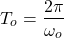#### Present Ampltiude

The signal's amplitude at present (time = to = 0) can be easily calculated using: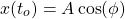because only the phase shift φ affects the present value.

#### Time Shift - Phase Shift Relationship

Time shifting a sinusoidal signal is related to phase shifting as follows:Thus, in this example, the time shift by to is equal to a phase shift by φ = ωoto.

Or, the other way around, a phase shift by any φ implies a time shift by some unknown multiple of ωo.

#### Even Sinusoidal

When the phase shift is φ = 0, the sinusoidal signal A cos ωot is falling into the even category.

For a signal to be even the following must be true: x(t) = x(-t), which is of course true for the cosine function, as:
#### Odd Sinusoidal

In a similar way, its also possible to prove that the sine function is odd, because: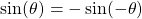Thus, the signal A sin ωot is considered an odd signal.

Its worth noting that the sine and cosine functions/signals differ by φ = -π/2. So, its easy to conclude that phase shifting by φ = -π/2, the cosine signal is also considered an odd signal: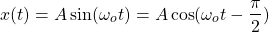### Discrete-Time Sinusoidals

In discrete-time, the sinusoidal signal is given by: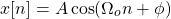where:

• A: amplitude
• Ωo: angular frequency
• φ: phase shift

#### Time Shift - Phase Shift Relationship

In the case of discrete-time, time shifting again implies a phase shift:
So, a time shift by no samples is equal to a phase shift by Ωono.

Let's note that phase shifting now doesn't imply a time shift, as the sample rate affects the outcome of phase shifting. As a result: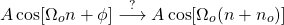#### Even-Odd Sinusoidal

Similar to continuous-time, the cosine signal is again considered an even signal, whilst the sine signal an odd signal:#### Requirements for Periodicity

Any sinusoidal signal is considered an periodic signal in continuous-time, but in discrete-time things change slightly.

In general, in discrete-time a signal is considered periodic only when their exists a small integer N for which: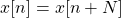In discrete-time, a sinusoidal signal: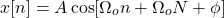is periodic when ΩoN is a multiple of , and so the following is true: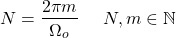The period N is the smallest natural number for which this equation is true. If none exists then the sinusoidal is aperiodic.

The following is a visualization of how the sample-rate affects the periodicity of a sinusoidal signal:[Image 3]

### Sinusoidals in Continuous- and Discrete-time at Distinct Frequencies

In addition to the issue of periodicity, continuous- and discrete-time sinusoidal also differ in other aspects.

In continuous-time, distinct values of the frequency ωo result into completely distinct signals. However, in discrete-time, values of Ωo which are separated by result into identical signals.

So, in continuous-time, if ω2 ≠ ω1 then x2(t) ≠ x1(t). But, in discrete-time, if Ω2 = Ω1 + 2πm then x2[n] ≠ x1[n].

## Exponential Signals

Exponential signals can be defined as: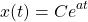where both C and a are real numbers.

### Time Shift - Scale Change Relationship

Time shifting an exponential signal implies scale change, as follows:### Complex Exponentials

Replacing C and a with complex numbers results in complex explonentials, which can be easily related to sinusoidal signals using Euler's relation.

#### Continuous-Time

In continuous-time, an complex exponential is defined as:where C and a tend to be defined as: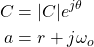which results in the following representation for complex exponentials: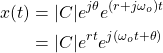Euler's relation allows us to replace the second exponential with a sum of cosine and sine: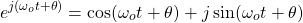And so, the final representation of complex exponentials is: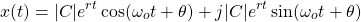where the real and imaginary parts are clearly separated.

Complex exponentials can be thought of as exponentially growing or decaying sinusoidal signals, as shown below.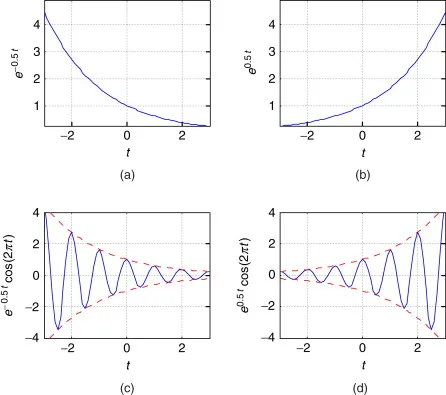[Image 4]

#### Discrete-Time

In discrete-time, an complex exponential is defined as: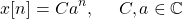where C and a are defined as: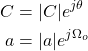therefore resulting into the following representation: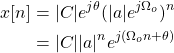Using Euler's relation, the last exponential can be replaced by sinusoidal signals, giving us the following, now final, form: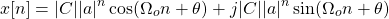## RESOURCES:

### Images

1.2.3.4. https://www.sciencedirect.com/topics/computer-science/complex-exponential

## Final words | Next up

And this is actually it for today's post! Till next time!

See Ya!Keep on drifting!

H2
H3
H4
3 columns
2 columns
1 column
1 Comment